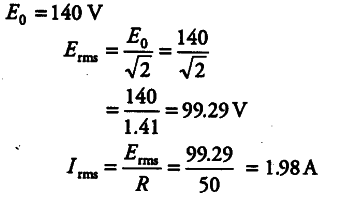# An alternating voltage given by E = 140 sin 314t

An alternating voltage given by E = 140 sin 314t is connected across a pure resistor of 50 Ω. Find
(i) the frequency of the source.
(ii) the rms current through the resistor.

(i)As, given E = 140 sin 314 t
On comparing with E = { E }_{ 0 } sin ω t , we have,
ω = 314, { E }_{ 0 } = 140V
ω = 2 \pi v
v = ω / 2 \pi = 314 / 2 x 3.14
= 31400/ 2 x 314 = 100/2 = 50Hz
(ii)